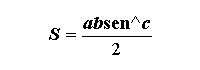Mathematics

Elements of a Triangle

 Show figure Calculate:   Area of the Triangle (S) Perimeter (p) Side a Side b Side c Angle ^a Angle ^b Angle ^c Radius of the Inscribed Circle (r) Radius of the Circumscribed Circle (R) 1- Side a:2- Side b: 3- Side c: 4- Angle ^a: º5- Angle ^b: º 6- Angle ^c: º 7- Height (h):8- Radius (r): 9- Radius (R): To Memory 01 Memory 02 Memory 03 Memory 04 Memory 05 Memory 06 Replacing Adding Subtracting Multiplying Dividing
 Configurations Maximum of decimal places Unit of Measurement of Length: Impression Page Title (Optional) Comments to be printed (Optional)